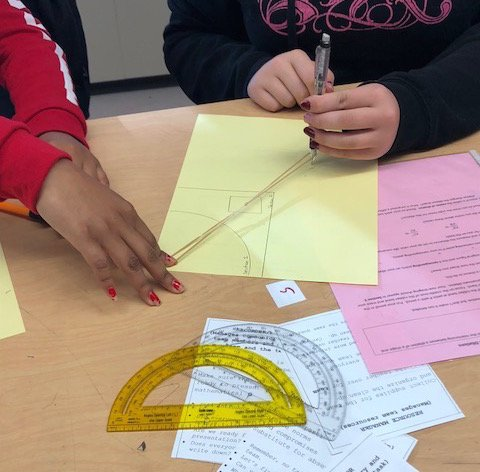# Geometry## Overview of Geometry

For the CCSS Geometry course, instructional time should focus on seven critical areas:

1. apply linear equations and the Pythagorean Theorem to the coordinate plane
2. establish criteria for congruence of triangles based on rigid motions;
3. establish criteria for similarity of triangles based on dilations and proportional reasoning;
4. prove theorems using logical arguments;
5. informally develop explanations of circumference, area, and volume formulas;
6. Identify relationships in circles and prove them; and
7. extend work with probability.